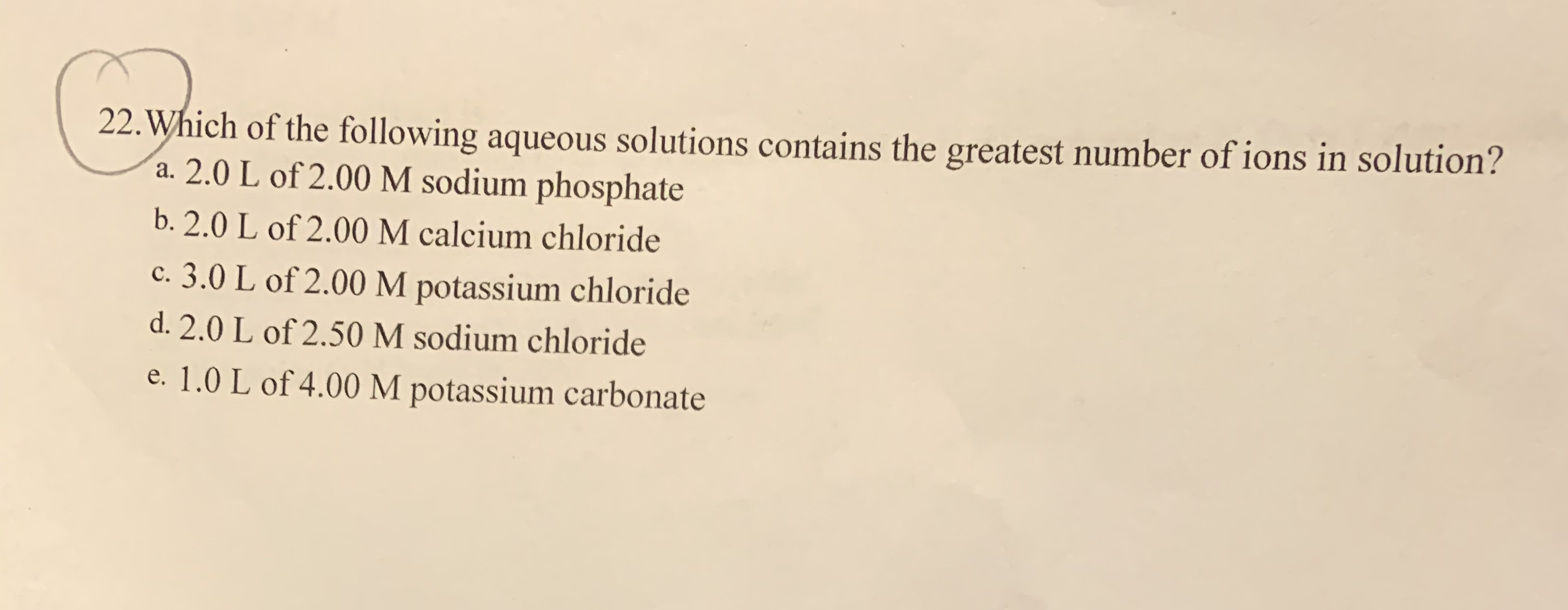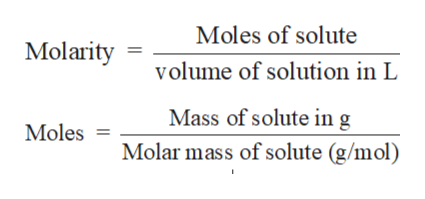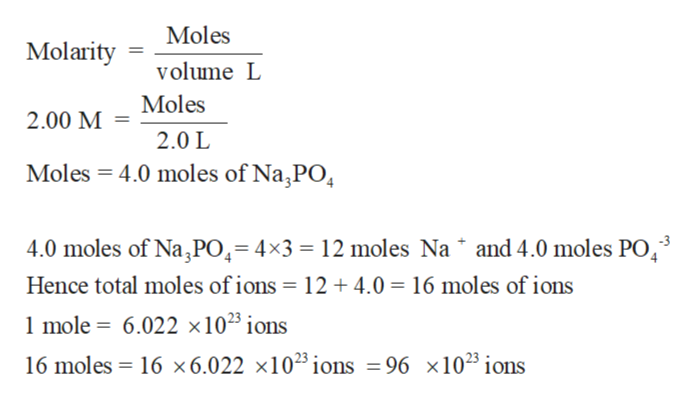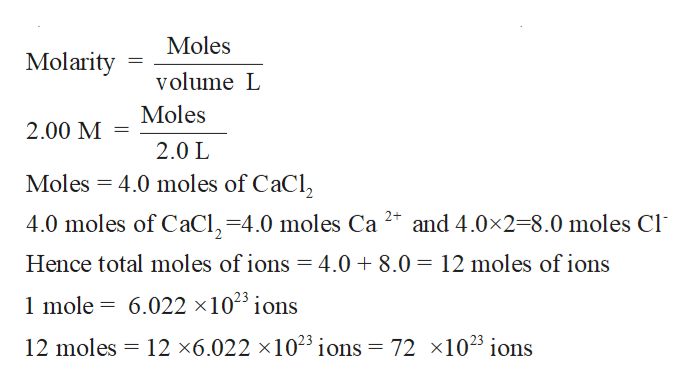# 22. Which of the following aqueous solutions contains the greatest number of ions in solution?a. 2.0 L of 2.00 M sodium phosphateb. 2.0 L of 2.00 M calcium chloridec. 3.0 L of 2.00 M potassium chlorided. 2.0 L of 2.50 M sodium chloridee. 1.0 L of 4.00 M potassium carbonate

Question
78 viewshelp_outlineImage Transcriptionclose22. Which of the following aqueous solutions contains the greatest number of ions in solution? a. 2.0 L of 2.00 M sodium phosphate b. 2.0 L of 2.00 M calcium chloride c. 3.0 L of 2.00 M potassium chloride d. 2.0 L of 2.50 M sodium chloride e. 1.0 L of 4.00 M potassium carbonate fullscreen
check_circle

Step 1

Solution stoichiometry involves the calculation of concentration of solutions in the given conditions of volumes, moles etc.

Solution stoichiometry is mainly based on the calculation of moles and volumes. These two values are used to calculate the molarity of solution. The relation between moles, volume and molarity is as given below;help_outlineImage TranscriptioncloseMoles of solute Molarity volume of solution in L Mass of solute in g Moles Molar mass of solute (g/mol) fullscreen
Step 2

a) 2.0 L of 2.00 M of sodium phosphate (Na3PO4)help_outlineImage TranscriptioncloseMoles Molarity volume L Moles 2.00 M = 2.0 L Moles = 4.0 moles of Na,PO, 4.0 moles of Na PO,=4×3 = 12 moles Na * and 4.0 moles PO,3 Hence total moles of ions = 12 + 4.0 = 16 moles of ions 1 mole = 6.022 ×10²³ ions 16 moles = 16 ×6.022 ×1023ions =96 ×1023 ions fullscreen
Step 3

b) 2.0 L of 2.00 M of calcium ch...help_outlineImage TranscriptioncloseMoles Molarity volume L Moles 2.00 M = 2.0 L Moles = 4.0 moles of CaCl, 4.0 moles of CaCl,=4.0 moles Ca 2* and 4.0x2=8.0 moles Cl Hence total moles of ions = 4.0 + 8.0 = 12 moles of ions 1 mole = 6.022 ×103 ions 12 moles = 12 ×6.022 ×1023ions = 72 ×1023 ions fullscreen

### Want to see the full answer?

See Solution

#### Want to see this answer and more?

Solutions are written by subject experts who are available 24/7. Questions are typically answered within 1 hour.*

See Solution
*Response times may vary by subject and question.
Tagged in

### Other